# Quiz 1: Marketings Value to Consumers, Firms, and Society

a. Following values are provided in the spreadsheet: Selling price (calendar) = 5 Quantity sold (calendar) = 90 percent of 3,000 = 2,700 Total cost (calendar) = 11,500 Selling price (notebook) = 2 Quantity sold (notebook) = 6,000 Total cost (notebook) = 9,000 Calculate the revenue and profit of Person S earned from the calendars and notebook using spreadsheet as shown below: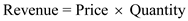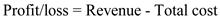Prepare a spreadsheet using given formulas: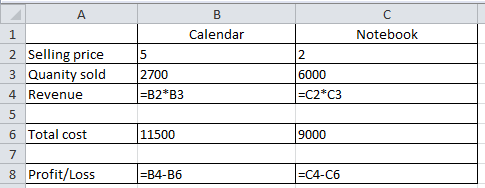It would give the following results: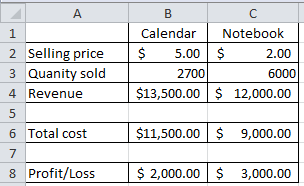Hence, the revenue for Person S from calendars and notebooks are \$13,500 and 12,000 , respectively. Profit earned from calendars and notebooks are \$2,000 and 3,000 , respectively. b. Calculate the expected revenue and profit for Person S after increasing the price of calendars to \$6.00, as shown below: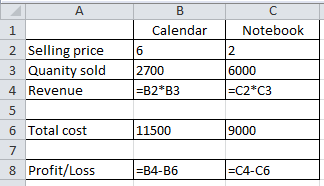It would give the following results: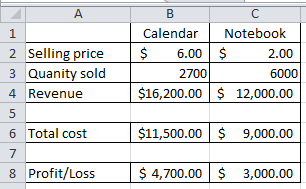Hence, increasing the price of calendars to \$6.00 would result in expected revenue of \$16,200. Expected profit after increasing the price of calendars is \$4,700. Now, perform the calculations manually to verify the results, as shown below:c. Prepare an excel sheet using the mentioned values and formulas, as shown below: Quantity sold = 6,000 Selling price = 1.60 - 2.40 (with a price difference of 8 cents) Total cost = 9,000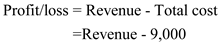Prepare an excel sheet as shown below: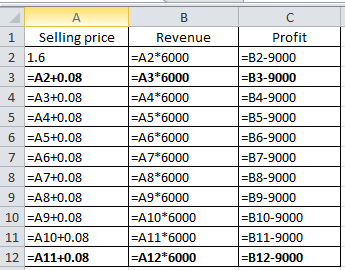It would give the following results: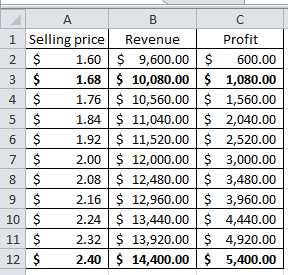From the above calculations, it is clear that selling \$6,000 notebooks at \$2.40 would contribute \$5,400 to profit. At a price of \$1.68 , selling 600 notebooks would contribute to the profit of \$1,080.00. Now, use "Goal Seek" analysis to obtain the required values, as shown below: Prepare an excel sheet as shown below: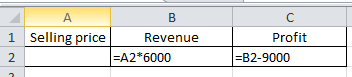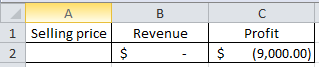Access "Goal Seek" option from "What if" analysis present under data menu bar, as shown below: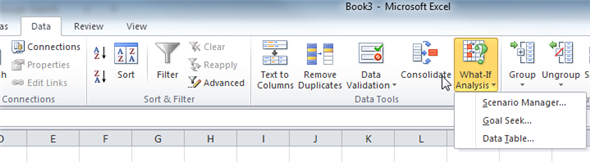Now, fill the following values in the "Goal Seek" window to calculate the selling price required to earn a profit of \$5,400.00: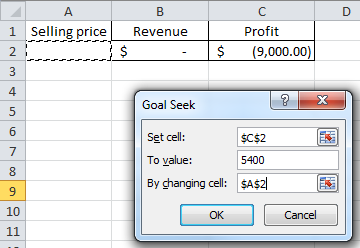It would give the following results: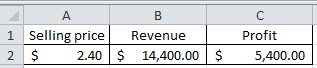Hence, selling price of \$2.40 would provide an expected profit of \$5,400.00 to Person S. Similarly, fill the following values in the "Goal Seek" window to calculate the selling price required to earn a profit of \$5,400.00: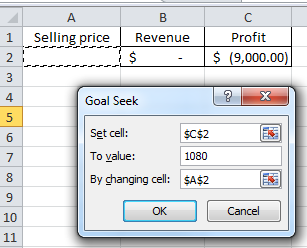It would give the following results: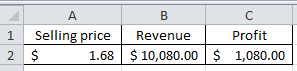Hence, selling price of \$1.68 would provide an expected profit of \$1,080.00 to Person S.